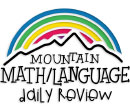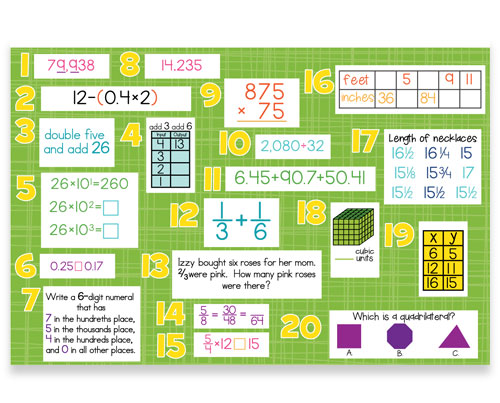WHAT YOU GET:
• 32 WEEKS OF DAILY REVIEW
• INSTRUCTIONS
• ONE MASTER STUDENT RESPONSE SHEET
• PRINTED ON HIGH-QUALITY CARD STOCK
• KNOWLEDGEABLE STAFF FOR ANY ASSISTANCE

This 5th GRADE COMMON CORE DAILY REVIEW MATH BULLETIN BOARD KIT is the DAILY REVIEW your students need to MASTER the concepts you have taught.

Research has proven that LONG-TERM MASTERY comes from SPIRAL REVIEW, also called DISTRIBUTED PRACTICE and SPACED REPETITION.

The Bulletin Board Kit is designed for total classroom viewing. It hangs on bulletin boards, walls, white boards, pocket charts, and science display boards. Once hung, it can remain displayed for the entire year. The cards can be interchanged, creating flexible and adjustable DAILY REVIEW.
1. 5.NBT.A.1- Recognize that in a multi-digit number, a digit in one place represents 10 times as much as it represents in the place to its right and 1/10 of what it represents in the place to its left.
5.NBT.A.2- Explain patterns in the number of zeros of the product when multiplying a number by powers of 10, and explain patterns in the placement of the decimal point when a decimal is multiplied or divided by a power of 10. Use whole-number exponents to denote powers of 10.
5.NBT.A.4- Use place value understanding to round decimals to any place.
2. 5.OA.A1- Use parentheses, brackets, or braces in numerical expressions, and evaluate expressions with these symbols.
3. 5.OA.A2- Write simple expressions that record calculations with numbers, and interpret numerical expressions without evaluating them.
4. 5.OA.B.3- Generate two numerical patterns using two given rules. Identify apparent relationships between corresponding terms. Form ordered pairs consisting of corresponding terms from the two patterns, and graph the ordered pairs on a coordinate plane.
5. 5.NBT.A.1- Recognize that in a multi-digit number, a digit in one place represents 10 times as much as it represents in the place to its right and 1/10 of what it represents in the place to its left.
5.NBT.A.2- Explain patterns in the number of zeros of the product when multiplying a number by powers of 10, and explain patterns in the placement of the decimal point when a decimal is multiplied or divided by a power of 10. Use whole-number exponents to denote powers of 10.
6. 5.NBT.A.3- Read, write, and compare decimals to thousandths.
7. 5.NBT.A.3- Read, write, and compare decimals to thousandths.
8. 5.NBT.A.4- Use place value understanding to round decimals to any place.
9. 5.NBT.B.5- Fluently multiply multi-digit whole numbers using the standard algorithm.
10. 5.NBT.B.6- Find whole-number quotients of whole numbers with up to four-digit dividends and two-digit divisors, using strategies based on place value, the properties of operations, and/or the relationship between multiplication and division. Illustrate and explain the calculation by using equations, rectangular arrays, and/or area models.
11. 5.NBT.B.7- Add, subtract, multiply, and divide decimals to hundredths, using concrete models or drawings and strategies based on place value, properties of operations, and/or the relationship between addition and subtraction; relate the strategy to a written method and explain the reasoning used.
12. 5.NF.A.1- Add and subtract fractions with unlike denominators (including mixed numbers) by replacing given fractions with equivalent fractions in such a way as to produce an equivalent sum or difference of fractions with like denominators.
13. 5.NF.A.2- Solve word problems involving addition and subtraction of fractions referring to the same whole, including cases of unlike denominators.
5.NF.A.3- Interpret a fraction as division of the numerator by the denominator. Solve word problems involving division of whole number leading to answers in the form of fractions or mixed numbers.
5.NF.A.6- Solve real world problems involving multiplication of fractions and mixed numbers.
5.NF.A.7- Apply and extend previous understandings of division to divide unit fractions by whole numbers and whole numbers by unit fractions.
14. 5.NF.A.4- Apply and extend previous understandings of multiplication to multiply a fraction or whole number by a fraction.
15. 5.NF.B.5- Interpret multiplication as scaling.
16. 5.MD.A.1- Convert among different-sized standard measurement units within a given measurement system, and use these conversions in solving multi-step, real world problems.
17. 5.MD.B.2- Make a line plot to display a data set of measurements in fractions of a unit. Use operations of fractions for this grade to solve problems involving information presented in line plots.
18. 5.MD.C.3- Recognize volume as an attribute of solid figures and understand concepts of volume and measurement.
5.MD.C.4- Measure volumes by counting unit cubes, using cubic cm, cubic in, cubic ft, and improvised units.
5.MD.C.5- Relate volume to the operations of multiplication and addition and solve real world and mathematical problems involving volume.
19. 5.G.A.1- Use a pair of perpendicular number lines, called axes, to define a coordinate system, with the intersection of lines (the origin) arranged to coincide with the O on each line and a given point into the plane located by using an ordered pair of numbers, called its coordinates.
5.G.A.2- Represent real world and mathematical problems by graphing points in the first quadrant of the coordinate plane, and interpret coordinate values of points in the context of the situation.
20. 5.G.B.3- Understand that attributes belonging to a category of two-dimensional figures also belong to all subcategories of that category.
5.G.B.4- Classify two-dimensional figures in a hierarchy based on properties.
ONLINE REVIEW VS.
CENTER EDITION VS.
BULLETIN BOARD KIT
ONLINE REVIEW
Is projected onto an interactive white board, creating a virtual board. It can be viewed by the entire classroom. Answers can be displayed onto the board at the click of a button. Up to seven questions can be isolated and magnified.

CENTER EDITION
Is organized on a 13"x19" flip chart. It can be viewed by 5-6 students at a time. It was designed for small group review, such as rotations, scoots, centers, or math-around-the-classroom. It has an answer key, heavy-duty stand, and high-gauged coil.

BULLETIN BOARD KIT
It is designed for total classroom view. It hangs on bulletin boards, walls, white boards, pocket charts, and science display boards. Once hung, it can remain displayed for the entire year. The cards can be interchanged, creating flexible and adjustable DAILY REVIEW.

\$95.95 QTY:
Bulletin Boards   |   5th Grade Common Core Math
To preview a grade level, click on the grade icon.

This will display covered concepts, images, and more.

## What teachers are saying about Mountain Math and Mountain Language:

"Hello! Thank you for asking how I like Mountain Math online. I love it! I also tried my fellow teacher's Mountain Language. I want it! I plan to purchase both for online use next year. I used the paper Mountain Math bulletin board last year, but relocated to a new school and had to leave it behind. I also plan to get ...(more)"
-Patty Simmons